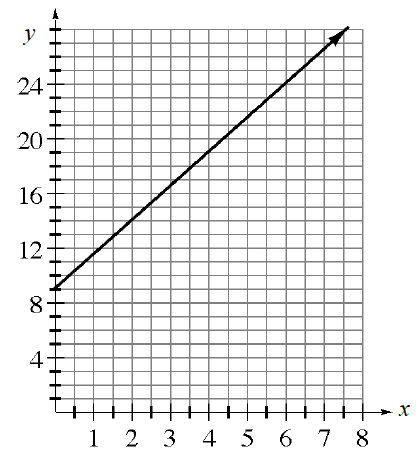### Home > GB8I > Chapter 5 Unit 6 > Lesson INT1: 5.3.1 > Problem5-91

5-91.

This problem is a checkpoint for writing the equation of a line. It will be referred to as Checkpoint 5.Use the given information to write the equation of each line.

1. Has a slope of $2$ and passing through $(10, 17)$.

2. Passing through $(1, –4)$ and $(–2, 5)$.

3.  $x$ $y$ $-6$ $-3$ $0$ $3$ $6$ $-6$ $-4$ $-2$ $0$ $2$
4. The line graphed below.Check your answers by referring to the Checkpoint 5 materials located at the back of your book.

Ideally, at this point you are comfortable working with these types of problems and can solve them correctly. If you feel that you need more confidence when solving these types of problems, then review the Checkpoint 5 materials and try the practice problems provided. From this point on, you will be expected to do problems like these correctly and with confidence.

Answers and extra practice are located in the back of your printed textbook or in the Reference Tab of your eBook. If you have an eBook for INT1, login and then click the following link: